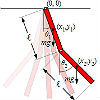# Finding Equations of Motion for Rigid Body Rotation

« Previous | Next »

## Session OverviewCovered this week: Week 7 emphasizes finding the equations of motion of rotating and translating rigid bodies. We introduce more complex problems and consider systems with more than one degree of freedom. We discuss how to cleverly select the point about which one computes torques and angular momentum and present examples. We use an important alternative form of Euler’s torque equation. Diagram of a double pendulum, a classic and extremely complex example of a system with multiple degrees of freedom. Public domain image.

## Assignments

### Problems and Concept Questions

Each problem set has concept questions paired with most problems. Answers to the concept questions are handed in before the overall problem set is due. You should therefore answer the concept questions in each problem set while watching the video lectures for the week. Then, use the Concept Question Answer Key (below in the Check Yourself section) to check your work before continuing to work on the problem set.

Please note that if your answer to the concept question is incorrect, it is likely that you may start down an unproductive pathway to a solution. Try to fully understand the answer to the concept question before you begin the regular problem.

## Check Yourself

### Concept Question Answer Key and Problem Set Solutions

The solutions are presented in two files, one with the answers to the concept questions, and one with solutions and in-depth explanations for the problems. Work the problems on your own and check your answers when you’re done.

Looking for something specific in this course? The Resource Index compiles links to most course resources in a single page.

« Previous | Next »

#### Learning Resource Types

theaters Lecture Videos
assignment_turned_in Problem Sets with Solutions
grading Exams with Solutions
theaters Recitation Videos
notes Lecture Notes